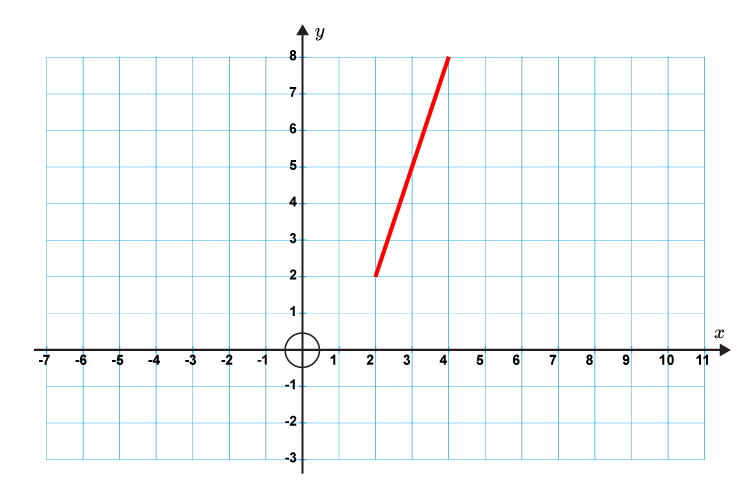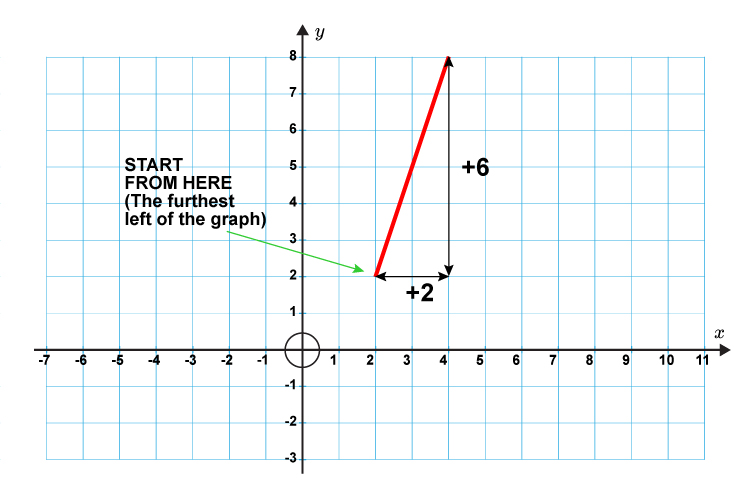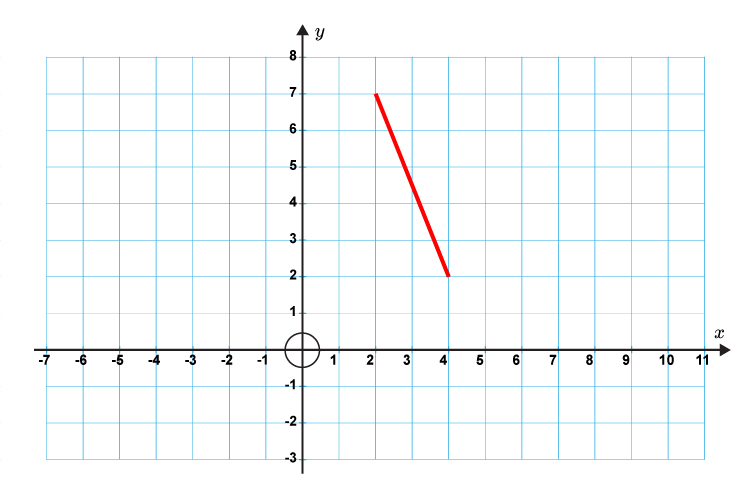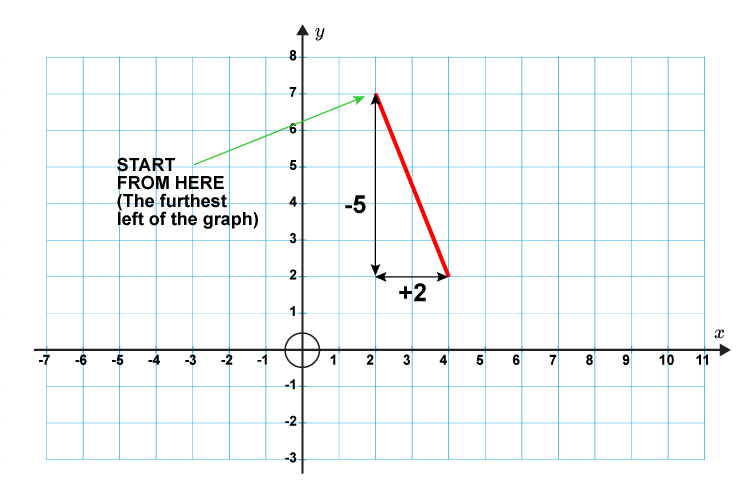# Further explanation

 Gradients UPHILL is POSITIVE DOWNHILL is NEGATIVE

But you don’t need to remember this because it becomes obvious when you start to measure from the left hand side.

Example

Work out the gradient of the following straight lines.Where do we start from?Gradient\ (m)=(y(chang\e\ i\n))/(x(chang\e\ i\n)

Gradient\ (m)=6/2=3

Example

Work out the gradient of the following straight lines.First, work out where we start from, then work out the horizontal (x)  and vertical (y)  points.Gradient(m)=(y(chang\e\ i\n))/(x(chang\e\ i\n)

Gradient(m)=(-5)/2=-2.5